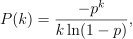/NumPy 1.14

# numpy.random.logseries

numpy.random.logseries(p, size=None)

Draw samples from a logarithmic series distribution.

Samples are drawn from a log series distribution with specified shape parameter, 0 < p < 1.

Parameters: p : float or array_like of floats Shape parameter for the distribution. Must be in the range (0, 1). size : int or tuple of ints, optional Output shape. If the given shape is, e.g., (m, n, k), then m * n * k samples are drawn. If size is None (default), a single value is returned if p is a scalar. Otherwise, np.array(p).size samples are drawn. out : ndarray or scalar Drawn samples from the parameterized logarithmic series distribution.

See also

scipy.stats.logser
probability density function, distribution or cumulative density function, etc.

#### Notes

The probability density for the Log Series distribution iswhere p = probability.

The log series distribution is frequently used to represent species richness and occurrence, first proposed by Fisher, Corbet, and Williams in 1943 . It may also be used to model the numbers of occupants seen in cars .

#### References

 [R475478] Buzas, Martin A.; Culver, Stephen J., Understanding regional species diversity through the log series distribution of occurrences: BIODIVERSITY RESEARCH Diversity & Distributions, Volume 5, Number 5, September 1999 , pp. 187-195(9).
 [R476478] Fisher, R.A,, A.S. Corbet, and C.B. Williams. 1943. The relation between the number of species and the number of individuals in a random sample of an animal population. Journal of Animal Ecology, 12:42-58.
 [R477478] D. J. Hand, F. Daly, D. Lunn, E. Ostrowski, A Handbook of Small Data Sets, CRC Press, 1994.
 [R478478] Wikipedia, “Logarithmic distribution”, http://en.wikipedia.org/wiki/Logarithmic_distribution

#### Examples

Draw samples from the distribution:

>>> a = .6
>>> s = np.random.logseries(a, 10000)
>>> count, bins, ignored = plt.hist(s)


# plot against distribution

>>> def logseries(k, p):
...     return -p**k/(k*log(1-p))
>>> plt.plot(bins, logseries(bins, a)*count.max()/
logseries(bins, a).max(), 'r')
>>> plt.show()


© 2008–2017 NumPy Developers
Licensed under the NumPy License.
https://docs.scipy.org/doc/numpy-1.14.2/reference/generated/numpy.random.logseries.html